set.seed(12345) # for reproducibility
options(knitr.kable.NA = '')

# Some packages need to be loaded. We use pacman as a package manager, which takes care of the other packages.
if (!require("pacman", quietly = TRUE)) install.packages("pacman")
if (!require("Rmisc", quietly = TRUE)) install.packages("Rmisc") # Never load it directly.
pacman::p_load(tidyverse, knitr, car, afex, emmeans, parallel, ordinal,
ggbeeswarm, RVAideMemoire)

klippy::klippy()

# 1 Item Repetition Phase

Twenty four participants were recruited. Half of the participants were exposed to 24 famous faces while the other half were exposed to 24 non-famous faces (pre-experimental stimulus familiarity as a between-participants factor).1 Each face was repeated eight times, resulting in 192 trials in total. For each face, participants made a male/female judgment.

P1 <- read.csv("data/data_FamSM_Exp1_Face_REP.csv", header = T)
P1$Familiarity = factor(P1$Familiarity, levels=c(1,2), labels=c("Famous","Non-famous"))

glimpse(P1, width=70)
## Observations: 4,608
## Variables: 9
## $SID <int> 1, 1, 1, 1, 1, 1, 1, 1, 1, 1, 1, 1, 1, 1, 1, 1,… ##$ Familiarity <fct> Famous, Famous, Famous, Famous, Famous, Famous,…
## $RepTime <int> 1, 1, 1, 1, 1, 1, 1, 1, 1, 1, 1, 1, 1, 1, 1, 1,… ##$ Trial       <int> 1, 2, 3, 4, 5, 6, 7, 8, 9, 10, 11, 12, 13, 14, …
## $ImgCat <int> 1, 2, 1, 2, 1, 1, 1, 2, 2, 2, 1, 2, 1, 2, 2, 1,… ##$ Resp        <int> 1, 2, 1, 1, 1, 1, 1, 2, 2, 2, 1, 2, 1, 2, 2, 1,…
## $Corr <int> 1, 1, 1, 0, 1, 1, 1, 1, 1, 1, 1, 1, 1, 1, 1, 1,… ##$ RT          <dbl> 477.59, 385.10, 337.84, 354.65, 395.43, 436.21,…
## $ImgName <fct> actor17.jpg, famfemale30.jpg, actor51.jpg, famf… # 1. SID: participant ID # 2. Familiarity: pre-experimental familiarity. 1 = famous, 2 = non-famous # 3. RepTime: number of repetitions, 1~8 # 4. Trial: 1~24 # 5. ImgCat: stimulus category. male vs. female # 6. Resp: male/female judgment, 1 = male, 2 = female, 0 = no response # 7. Corr: correctness, 1=correct, 0 = incorrect or no response # 8. RT: reaction times in ms. # 9. ImgName: name of stimuli table(P1$Familiarity, P1$SID) ## ## 1 2 3 4 5 6 7 8 9 10 11 12 13 14 15 ## Famous 192 192 192 192 192 192 192 192 192 192 192 192 0 0 0 ## Non-famous 0 0 0 0 0 0 0 0 0 0 0 0 192 192 192 ## ## 16 17 18 19 20 21 22 23 24 ## Famous 0 0 0 0 0 0 0 0 0 ## Non-famous 192 192 192 192 192 192 192 192 192 ## 1.1 Accuracy We calculated the mean and s.d. of individual participants’ mean percentage accuracy. Overall accuracy of the male/female judgment was high. The famous condition showed lower mean accuracy and larger variance than the non-famous condition. In the following plot, red points and error bars represent the means and 95% CIs. # phase 1, subject-level, long-format P1slong <- P1 %>% group_by(SID, Familiarity) %>% summarise(Accuracy = mean(Corr)*100) %>% ungroup() # summary table P1slong %>% group_by(Familiarity) %>% summarise(M = mean(Accuracy), SD = sd(Accuracy)) %>% ungroup() %>% kable() Familiarity M SD Famous 98.26389 1.301294 Non-famous 95.31250 6.269698 # group level, needed for printing & geom_pointrange # Rmisc must be called indirectly due to incompatibility between plyr and dplyr. P1g <- Rmisc::summarySE(data = P1slong, measurevar = "Accuracy", groupvars = "Familiarity") ggplot(P1slong, aes(x=Familiarity, y=Accuracy)) + geom_violin(width = 0.5, trim=TRUE) + ggbeeswarm::geom_quasirandom(color = "blue", size = 3, alpha = 0.2, width = 0.2) + geom_pointrange(P1g, inherit.aes=FALSE, mapping=aes(x = Familiarity, y=Accuracy, ymin = Accuracy - ci, ymax = Accuracy + ci), colour="darkred", size = 1)+ coord_cartesian(ylim = c(50, 100), clip = "on") + labs(x = "Pre-experimental Stimulus Familiarity", y = "Male/Female Judgment \n Accuracy (%)") + theme_bw(base_size = 18)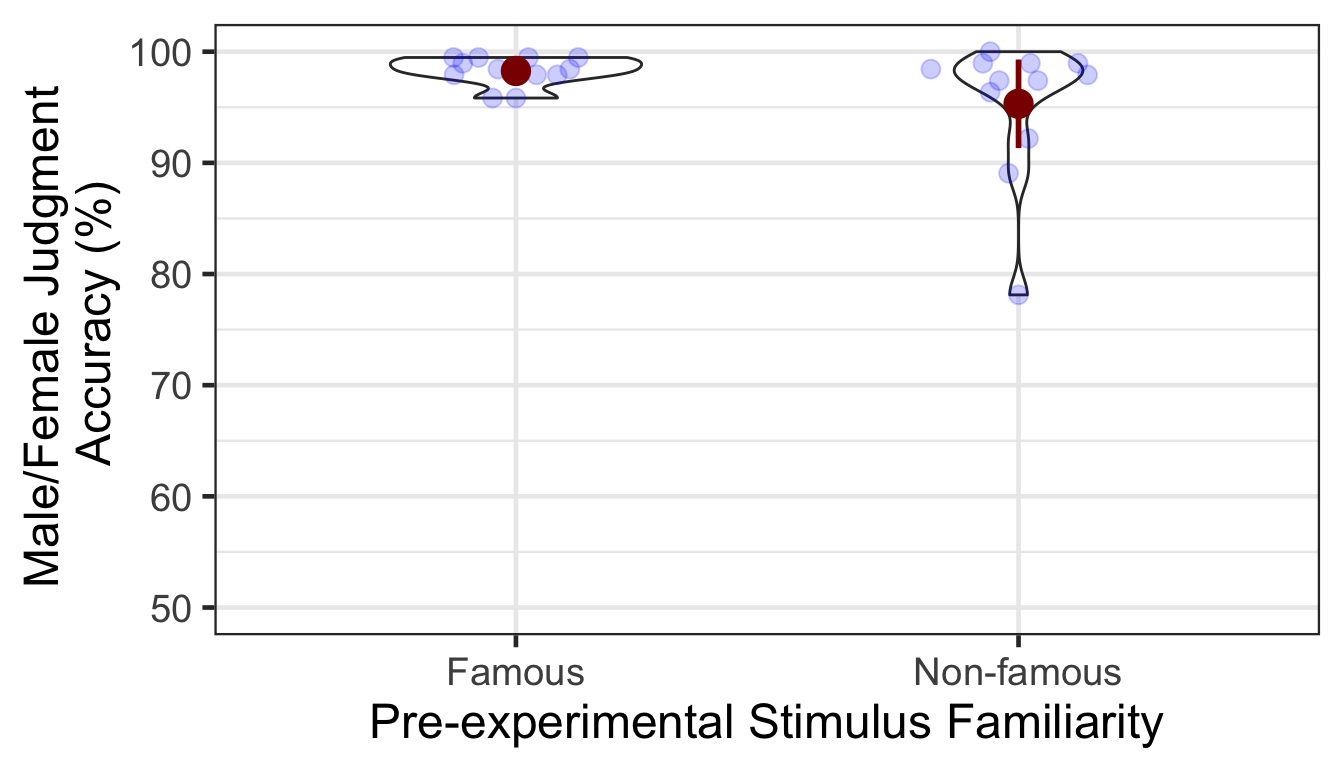### 1.1.1 ANOVA Levene’s test indicated that the assumption of homogeneity of variance was not violated. Then, a one-way ANOVA showed that the difference in accuracy was not significant between famous vs. non-famous conditions. car::leveneTest(Accuracy ~ Familiarity, P1slong) ## Levene's Test for Homogeneity of Variance (center = median) ## Df F value Pr(>F) ## group 1 2.5262 0.1262 ## 22 p1.aov <- aov_ez(id = "SID", dv = "Accuracy", data = P1slong, between = "Familiarity") anova(p1.aov, es = "pes") %>% kable(digits = 4) num Df den Df MSE F pes Pr(>F) Familiarity 1 22 20.5012 2.5493 0.1038 0.1246 # 2 Item-Source Association Phase Participants learned 48 face-location (a quadrant on the screen) associations. They were instructed to pay attention to the location of each face while making a male/female judgment. A between-participants pre-experimental stimulus familiarity factor determined whether a participant viewed famous or non-famous faces. A within-participant item repetition factor determined whether a repeated item (which had been presented in the first phase) or an unrepeated item (which appeared for the first time in the item-source association phase) was presened in each trial. P2 <- read.csv("data/data_FamSM_Exp1_Face_SRC.csv", header = T) P2$Familiarity = factor(P2$Familiarity, levels=c(1,2), labels=c("Famous","Non-famous")) P2$Repetition = factor(P2$Repetition, levels=c(1,2), labels=c("Repeated","Unrepeated")) glimpse(P2, width=70) ## Observations: 1,152 ## Variables: 10 ##$ SID         <int> 1, 1, 1, 1, 1, 1, 1, 1, 1, 1, 1, 1, 1, 1, 1, 1,…
## $Familiarity <fct> Famous, Famous, Famous, Famous, Famous, Famous,… ##$ Trial       <int> 1, 2, 3, 4, 5, 6, 7, 8, 9, 10, 11, 12, 13, 14, …
## $Repetition <fct> Unrepeated, Unrepeated, Repeated, Repeated, Unr… ##$ ImgCat      <int> 1, 1, 2, 1, 1, 1, 2, 2, 2, 2, 2, 2, 2, 1, 1, 2,…
## $Loc <int> 3, 4, 3, 2, 3, 1, 2, 1, 4, 2, 3, 1, 3, 1, 1, 3,… ##$ Resp        <int> 1, 1, 2, 1, 1, 1, 2, 2, 2, 2, 2, 2, 2, 1, 1, 2,…
## $Corr <int> 1, 1, 1, 1, 1, 1, 1, 1, 1, 1, 1, 1, 1, 1, 1, 1,… ##$ RT          <dbl> 1135.99, 692.29, 536.67, 789.05, 1257.40, 789.7…
## $ImgName <fct> actor9.jpg, athlete13.jpg, famfemale01.jpg, act… # 1. SID: participant ID # 2. Familiarity: pre-experimental familiarity. 1 = famous, 2 = non-famous # 3. Trial: 1~48 # 4. Repetition: 1 = repeated, 2 = unrepeated # 5. ImgCat: stimulus category. male vs. female # 6. Loc: location (source) of memory item; quadrants, 1~4 # 7. Resp: male/female judgment, 1 = male, 2 = female, 0 = no response # 8. Corr: correctness, 1 = correct, 0 = incorrect or no response # 9. RT: reaction times in ms. # 10. ImgName: name of stimuli table(P2$Familiarity, P2$SID) ## ## 1 2 3 4 5 6 7 8 9 10 11 12 13 14 15 16 17 18 19 20 ## Famous 48 48 48 48 48 48 48 48 48 48 48 48 0 0 0 0 0 0 0 0 ## Non-famous 0 0 0 0 0 0 0 0 0 0 0 0 48 48 48 48 48 48 48 48 ## ## 21 22 23 24 ## Famous 0 0 0 0 ## Non-famous 48 48 48 48 table(P2$Repetition, P2$SID) ## ## 1 2 3 4 5 6 7 8 9 10 11 12 13 14 15 16 17 18 19 20 ## Repeated 24 24 24 24 24 24 24 24 24 24 24 24 24 24 24 24 24 24 24 24 ## Unrepeated 24 24 24 24 24 24 24 24 24 24 24 24 24 24 24 24 24 24 24 24 ## ## 21 22 23 24 ## Repeated 24 24 24 24 ## Unrepeated 24 24 24 24 ## 2.1 Accuracy We calculated the mean and s.d. of individual participants’ mean percentage accuracy. Overall accuracy was high. The non-famous, unrepeated condition showed slightly lower mean accuracy than the other conditions. # phase 2, subject-level, long-format P2slong <- P2 %>% group_by(SID, Familiarity, Repetition) %>% summarise(Accuracy = mean(Corr)*100) %>% ungroup() # summary table P2g <- P2slong %>% group_by(Familiarity, Repetition) %>% summarise(M = mean(Accuracy), SD = sd(Accuracy)) %>% ungroup() P2g %>% kable() Familiarity Repetition M SD Famous Repeated 97.22222 3.698439 Famous Unrepeated 98.61111 3.243746 Non-famous Repeated 98.26389 3.751403 Non-famous Unrepeated 94.44444 10.102588 # group level, needed for printing & geom_pointrange # Rmisc must be called indirectly due to incompatibility between plyr and dplyr. P2g$ci <- Rmisc::summarySEwithin(data = P2slong, measurevar = "Accuracy", idvar = "SID",
withinvars = "Repetition", betweenvars = "Familiarity")$ci P2g$Accuracy <- P2g$M ggplot(data=P2slong, aes(x=Familiarity, y=Accuracy, fill=Repetition)) + geom_violin(width = 0.7, trim=TRUE) + ggbeeswarm::geom_quasirandom(dodge.width = 0.7, color = "blue", size = 3, alpha = 0.2, show.legend = FALSE) + # geom_pointrange(data=P2g, # aes(x = Familiarity, ymin = Accuracy-ci, ymax = Accuracy+ci, color = Repetition), # position = position_dodge(0.7), color = "darkred", size = 1, show.legend = FALSE) + coord_cartesian(ylim = c(50, 100), clip = "on") + labs(x = "Pre-experimental Stimulus Familiarity", y = "Male/Female Judgment \n Accuracy (%)", fill="Item Repetition") + scale_fill_manual(values=c("#E69F00", "#56B4E9"), labels=c("Repeated", "Unrepeated")) + theme_bw(base_size = 18) + theme(panel.grid.major = element_blank(), panel.grid.minor = element_blank())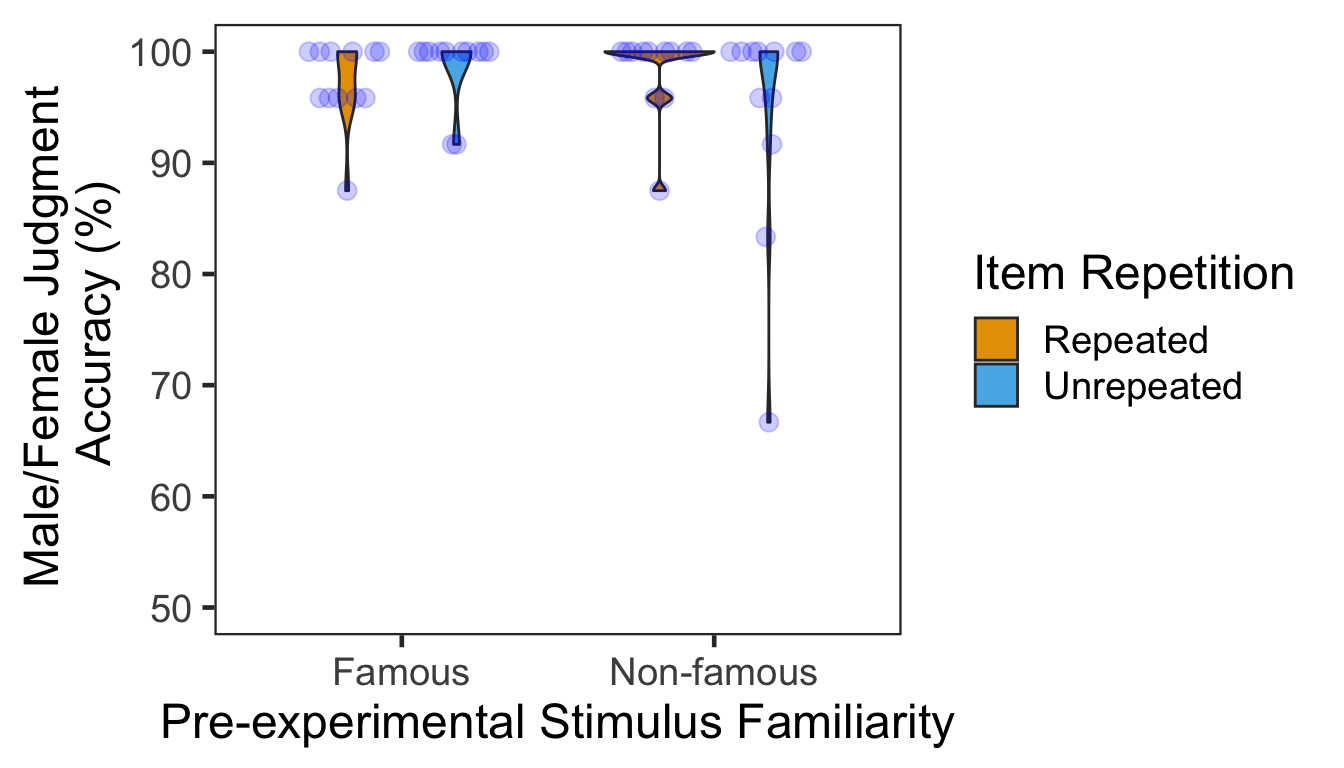### 2.1.1 ANOVA Mean percentage accuracy was submitted to a 2x2 mixed design ANOVA with pre-experimental stimulus familiarity as a between-participants factor and item repetition as a within-participant factor. Both main effects failed to reach significance. The two-way interaction was marginally significant. p2.aov <- aov_ez(id = "SID", dv = "Accuracy", data = P2slong, between = "Familiarity", within = "Repetition") anova(p2.aov, es = "pes") %>% kable(digits = 4) num Df den Df MSE F pes Pr(>F) Familiarity 1 22 49.8146 0.5881 0.0260 0.4513 Repetition 1 22 20.3533 0.8708 0.0381 0.3609 Familiarity:Repetition 1 22 20.3533 3.9984 0.1538 0.0580 # 3 Source Memory Test Phase In each trial, participants first indicated in which quadrant a given face appeared during the item-source association phase. Participants then rated how confident they were about their memory judgment. There were 48 trials in total. P3 <- read.csv("data/data_FamSM_Exp1_Face_TST.csv", header = T) P3$Familiarity = factor(P3$Familiarity, levels=c(1,2), labels=c("Famous","Non-famous")) P3$Repetition = factor(P3$Repetition, levels=c(1,2), labels=c("Repeated","Unrepeated")) glimpse(P3, width=70) ## Observations: 1,152 ## Variables: 10 ##$ SID         <int> 1, 1, 1, 1, 1, 1, 1, 1, 1, 1, 1, 1, 1, 1, 1, 1,…
## $Familiarity <fct> Famous, Famous, Famous, Famous, Famous, Famous,… ##$ Trial       <int> 1, 2, 3, 4, 5, 6, 7, 8, 9, 10, 11, 12, 13, 14, …
## $Repetition <fct> Repeated, Repeated, Unrepeated, Repeated, Unrep… ##$ AscLoc      <int> 1, 2, 3, 3, 2, 2, 3, 4, 1, 4, 2, 4, 2, 2, 3, 4,…
## $ScrResp <int> 1, 3, 1, 2, 3, 2, 1, 4, 1, 4, 3, 2, 2, 2, 3, 4,… ##$ Corr        <int> 1, 0, 0, 0, 0, 1, 0, 1, 1, 1, 0, 0, 1, 1, 1, 1,…
## $RT <dbl> 3065.59, 2146.18, 2411.76, 1512.09, 2817.44, 14… ##$ Confident   <int> 2, 2, 3, 3, 1, 4, 4, 4, 3, 4, 1, 3, 4, 1, 4, 2,…
## $ImgName <fct> famfemale21.jpg, athlete28.jpg, famfemale37.jpg… # 1. SID: participant ID # 2. Familiarity: pre-experimental familiarity. 1 = famous, 2 = non-famous # 3. Trial: 1~48 # 4. Repetition: 1 = repeated, 2 = unrepeated # 5. AscLoc: location (source) in which the item was presented in Phase 2; quadrants, 1~4 # 6. SrcResp: source response; quadrants, 1~4 # 7. Corr: correctness, 1=correct, 0=incorrect # 8. RT: reaction times in ms. # 9. Confident: confidence rating, 1~4 # 10. ImgName: name of stimuli table(P3$Familiarity, P3$SID) ## ## 1 2 3 4 5 6 7 8 9 10 11 12 13 14 15 16 17 18 19 20 ## Famous 48 48 48 48 48 48 48 48 48 48 48 48 0 0 0 0 0 0 0 0 ## Non-famous 0 0 0 0 0 0 0 0 0 0 0 0 48 48 48 48 48 48 48 48 ## ## 21 22 23 24 ## Famous 0 0 0 0 ## Non-famous 48 48 48 48 table(P3$Repetition, P3$SID) ## ## 1 2 3 4 5 6 7 8 9 10 11 12 13 14 15 16 17 18 19 20 ## Repeated 24 24 24 24 24 24 24 24 24 24 24 24 24 24 24 24 24 24 24 24 ## Unrepeated 24 24 24 24 24 24 24 24 24 24 24 24 24 24 24 24 24 24 24 24 ## ## 21 22 23 24 ## Repeated 24 24 24 24 ## Unrepeated 24 24 24 24 ## 3.1 Accuracy We calculated the mean and s.d. of individual participants’ mean percentage accuracy. In the following plot, red points and error bars represent the means and 95% within-participants CIs. # phase 3, subject-level, long-format P3ACCslong <- P3 %>% group_by(SID, Familiarity, Repetition) %>% summarise(Accuracy = mean(Corr)*100) %>% ungroup() # summary table P3ACCg <- P3ACCslong %>% group_by(Familiarity, Repetition) %>% summarise(M = mean(Accuracy), SD = sd(Accuracy)) %>% ungroup() P3ACCg %>% kable() Familiarity Repetition M SD Famous Repeated 48.95833 17.86638 Famous Unrepeated 65.27778 12.97498 Non-famous Repeated 43.75000 14.70458 Non-famous Unrepeated 34.72222 13.45275 # marginal means of famous vs. non-famous conditions. P3ACCslong %>% group_by(Familiarity) %>% summarise(M = mean(Accuracy), SD = sd(Accuracy)) %>% ungroup() %>% kable() Familiarity M SD Famous 57.11806 17.39700 Non-famous 39.23611 14.53365 # marginal means of repeated vs. unrepeated conditions. P3ACCslong %>% group_by(Repetition) %>% summarise(M = mean(Accuracy), SD = sd(Accuracy)) %>% ungroup() %>% kable() Repetition M SD Repeated 46.35417 16.22199 Unrepeated 50.00000 20.26396 # wide format, needed for geom_segments. P3ACCswide <- P3ACCslong %>% spread(key = Repetition, value = Accuracy) # group level, needed for printing & geom_pointrange # Rmisc must be called indirectly due to incompatibility between plyr and dplyr. P3ACCg$ci <- Rmisc::summarySEwithin(data = P3ACCslong, measurevar = "Accuracy", idvar = "SID",
withinvars = "Repetition", betweenvars = "Familiarity")$ci P3ACCg$Accuracy <- P3ACCg$M ggplot(data=P3ACCslong, aes(x=Familiarity, y=Accuracy, fill=Repetition)) + geom_violin(width = 0.5, trim=TRUE) + geom_point(position=position_dodge(0.5), color="gray80", size=1.8, show.legend = FALSE) + geom_segment(data=filter(P3ACCswide, Familiarity=="Famous"), inherit.aes = FALSE, aes(x=1-.12, y=filter(P3ACCswide, Familiarity=="Famous")$Repeated,
xend=1+.12, yend=filter(P3ACCswide, Familiarity=="Famous")$Unrepeated), color="gray80") + geom_segment(data=filter(P3ACCswide, Familiarity=="Non-famous"), inherit.aes = FALSE, aes(x=2-.12, y=filter(P3ACCswide, Familiarity=="Non-famous")$Repeated,
xend=2+.12, yend=filter(P3ACCswide, Familiarity=="Non-famous")$Unrepeated), color="gray80") + geom_pointrange(data=P3ACCg, aes(x = Familiarity, ymin = Accuracy-ci, ymax = Accuracy+ci, group = Repetition), position = position_dodge(0.5), color = "darkred", size = 1, show.legend = FALSE) + scale_fill_manual(values=c("#E69F00", "#56B4E9"), labels=c("Repeated", "Unrepeated")) + labs(x = "Pre-experimental Stimulus Familiarity", y = "Source Memory Accuracy (%)", fill='Item Repetition') + coord_cartesian(ylim = c(0, 100), clip = "on") + theme_bw(base_size = 18) + theme(panel.grid.major = element_blank(), panel.grid.minor = element_blank())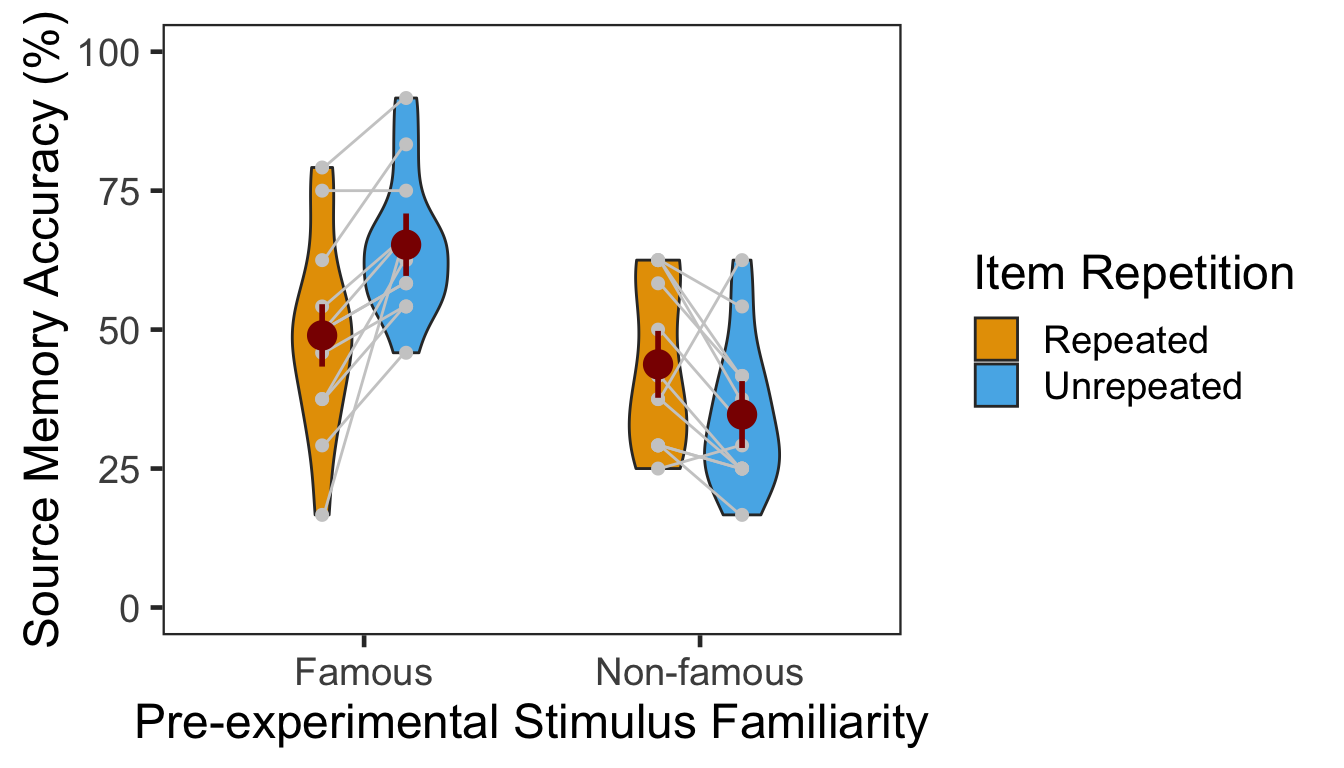The effect of item repetition was modulated by the pre-experimental stimulus familiarity of the items. For famous faces, the locations of unrepeated items were better remembered (novelty benefit). For non-famous faces, the locations of repeated items were better remembered (familiarity benefit). These crossed effects were on top of the general familiarity benefit, in which source memory was more accurate for famous faces than for non-famous faces. ### 3.1.1 ANOVA Mean percentage accuracy was submitted to a 2x2 mixed design ANOVA with pre-experimental stimulus familiarity as a between-participants factor (famous vs. non-famous) and item repetition as a within-participant factor (repeated vs. unrepeated). p3.corr.aov <- aov_ez(id = "SID", dv = "Accuracy", data = P3ACCslong, between = "Familiarity", within = "Repetition") anova(p3.corr.aov, es = "pes") %>% kable(digits = 4) num Df den Df MSE F pes Pr(>F) Familiarity 1 22 358.5004 10.7034 0.3273 0.0035 Repetition 1 22 83.8792 1.9016 0.0796 0.1818 Familiarity:Repetition 1 22 83.8792 22.9788 0.5109 0.0001 The main effect of pre-experimental stimulus familiarity and the two-way interaction were both significant. Additionally, we performed two separate one-way repeated-measures ANOVAs as post-hoc analyses. The table below shows the effect of item repetition for famous faces. ci95 <- P3ACCswide %>% filter(Familiarity=="Famous") %>% mutate(Diff = Unrepeated - Repeated) %>% summarise(lower = mean(Diff) - qt(0.975,df=n()-1)*sd(Diff)/sqrt(n()), upper = mean(Diff) + qt(0.975,df=n()-1)*sd(Diff)/sqrt(n())) p3.corr.aov.r1 <- aov_ez(id = "SID", dv = "Accuracy", within = "Repetition", data = filter(P3ACCslong, Familiarity == "Famous")) anova(p3.corr.aov.r1, es = "pes") %>% kable(digits = 4) num Df den Df MSE F pes Pr(>F) Repetition 1 11 78.0592 20.4709 0.6505 9e-04 In the famous face group, source memory was more accurate for unrepeated than repeated faces. The 95% CI of difference between the means was [8.38, 24.26]. The table below shows the effect of item repetition for non-famous faces. ci95 <- P3ACCswide %>% filter(Familiarity=="Non-famous") %>% mutate(Diff = Repeated - Unrepeated) %>% summarise(lower = mean(Diff) - qt(0.975,df=n()-1)*sd(Diff)/sqrt(n()), upper = mean(Diff) + qt(0.975,df=n()-1)*sd(Diff)/sqrt(n())) p3.corr.aov.r2 <- aov_ez(id = "SID", dv = "Accuracy", within = "Repetition", data = filter(P3ACCslong, Familiarity == "Non-famous")) anova(p3.corr.aov.r2, es = "pes") %>% kable(digits = 4) num Df den Df MSE F pes Pr(>F) Repetition 1 11 89.6991 5.4516 0.3314 0.0395 In the non-famous face group, source memory was more accurate for repeated than unrepeated faces. The 95% CI of difference between the means was [0.52, 17.54]. ### 3.1.2 GLMM To supplement conventional ANOVAs, we tested generalized linear mixed models (GLMM) on source memory accuracy. This mixed modeling approach with a binomial link function is expected to properly handle binary data such as source memory responses (i.e., correct or not; Jaeger, 2008). We built the full model (full1) with two fixed effects (pre-experimental stimulus familiarity and item repetition) and their interaction. The model also included maximal random effects structure (Barr, Levy, Scheepers, & Tily, 2013): both by-participant and by-item random intercepts, and by-participant random slopes for item repetition. In case the maximal model does not converge successfully, we built another model (full2) with the maximal random structure but with the correlations among the random terms removed (Singmann, 2018). To fit the models, we used the mixed() of the afex package (Singmann, Bolker, & Westfall, 2017) which was built on the lmer() of the lme4 package (Bates, Maechler, Bolker, & Walker, 2015). The mixed() assessed the statistical significance of fixed effects by comparing a model with the effect in question against its nested model which lacked the effect in question. P-values of the effects were obtained by likelihood ratio tests (LRT). (nc <- detectCores()) cl <- makeCluster(rep("localhost", nc)) full1 <- afex::mixed(Corr ~ Familiarity*Repetition + (Repetition|SID) + (1|ImgName), P3, method = "LRT", cl = cl, family=binomial(link="logit"), control = glmerControl(optCtrl = list(maxfun = 1e6))) full2 <- afex::mixed(Corr ~ Familiarity*Repetition + (Repetition||SID) + (1|ImgName), P3, method = "LRT", cl = cl, family=binomial(link="logit"), control = glmerControl(optCtrl = list(maxfun = 1e6)), expand_re = TRUE) stopCluster(cl) The table below presents the LRT results of the models full1 and full2. full.compare <- cbind(afex::nice(full1), afex::nice(full2)[,-c(1,2)]) colnames(full.compare)[c(3,4,5,6)] <- c("full1 Chisq", "p","full2 Chisq", "p") full.compare %>% kable() Effect df full1 Chisq p full2 Chisq p Familiarity 1 8.74 ** .003 8.81 ** .003 Repetition 1 1.49 .22 1.56 .21 Familiarity:Repetition 1 16.22 *** <.0001 17.48 *** <.0001 The p-values from the two models were highly similar to each other. Post-hoc analysis results are summarized in the table below. The results from the pairwise comparisons were consistent with those from the ANOVA. Item repetition impaired source memory for famous faces whereas it improved source memory for non-famous faces. emmeans(full1, pairwise ~ Repetition | Familiarity, type = "response")$contrasts %>% kable()
contrast Familiarity odds.ratio SE df z.ratio p.value
Repeated / Unrepeated Famous 0.4826584 0.0884641 Inf -3.974386 0.0000706
Repeated / Unrepeated Non-famous 1.5113764 0.2759630 Inf 2.262005 0.0236971

## 3.2 Confidence

The following table presents the mean and s.d. of individual participants’ confidence ratings in each condition. The pattern of confidence ratings was qualitatively identical to that of source memory accuracy: we observed the novelty benefit for famous faces and the familiarity benefit for non-famous faces. In the following plot, red points and error bars represent the means and 95% within-participants CIs.

P3CFslong <- P3 %>% group_by(SID, Familiarity, Repetition) %>%
summarise(Confidence = mean(Confident)) %>%
ungroup()

P3CFg <- P3CFslong %>%
group_by(Familiarity, Repetition) %>%
summarise(M = mean(Confidence), SD = sd(Confidence)) %>%
ungroup()
P3CFg %>% kable()
Familiarity Repetition M SD
Famous Repeated 2.437500 0.4555702
Famous Unrepeated 2.826389 0.4085703
Non-famous Repeated 2.625000 0.5047126
Non-famous Unrepeated 2.083333 0.4660169
# marginal means of famous vs. non-famous conditions.
P3CFslong %>% group_by(Familiarity) %>%
summarise(M = mean(Confidence), SD = sd(Confidence)) %>%
ungroup() %>% kable()
Familiarity M SD
Famous 2.631944 0.4674919
Non-famous 2.354167 0.5497584
# marginal means of repeated vs. unrepeated conditions.
P3CFslong %>% group_by(Repetition) %>%
summarise(M = mean(Confidence), SD = sd(Confidence)) %>%
ungroup() %>% kable()
Repetition M SD
Repeated 2.531250 0.4798554
Unrepeated 2.454861 0.5724814
# wide format, needed for geom_segments.
P3CFswide <- P3CFslong %>% spread(key = Repetition, value = Confidence)

# group level, needed for printing & geom_pointrange
# Rmisc must be called indirectly due to incompatibility between plyr and dplyr.
P3CFg$ci <- Rmisc::summarySEwithin(data = P3CFslong, measurevar = "Confidence", idvar = "SID", withinvars = "Repetition", betweenvars = "Familiarity")$ci
P3CFg$Confidence <- P3CFg$M

ggplot(data=P3CFslong, aes(x=Familiarity, y=Confidence, fill=Repetition)) +
geom_violin(width = 0.5, trim=TRUE) +
geom_point(position=position_dodge(0.5), color="gray80", size=1.8, show.legend = FALSE) +
geom_segment(data=filter(P3CFswide, Familiarity=="Famous"), inherit.aes = FALSE,
aes(x=1-.12, y=filter(P3CFswide, Familiarity=="Famous")$Repeated, xend=1+.12, yend=filter(P3CFswide, Familiarity=="Famous")$Unrepeated),
color="gray80") +
geom_segment(data=filter(P3CFswide, Familiarity=="Non-famous"), inherit.aes = FALSE,
aes(x=2-.12, y=filter(P3CFswide, Familiarity=="Non-famous")$Repeated, xend=2+.12, yend=filter(P3CFswide, Familiarity=="Non-famous")$Unrepeated),
color="gray80") +
geom_pointrange(data=P3CFg,
aes(x = Familiarity, ymin = Confidence-ci, ymax = Confidence+ci, group = Repetition),
position = position_dodge(0.5), color = "darkred", size = 1, show.legend = FALSE) +
scale_fill_manual(values=c("#E69F00", "#56B4E9"),
labels=c("Repeated", "Unrepeated")) +
labs(x = "Pre-experimental Stimulus Familiarity",
y = "Source Memory Confidence",
fill='Item Repetition') +
coord_cartesian(ylim = c(1, 4), clip = "on") +
theme_bw(base_size = 18) +
theme(panel.grid.major = element_blank(),
panel.grid.minor = element_blank())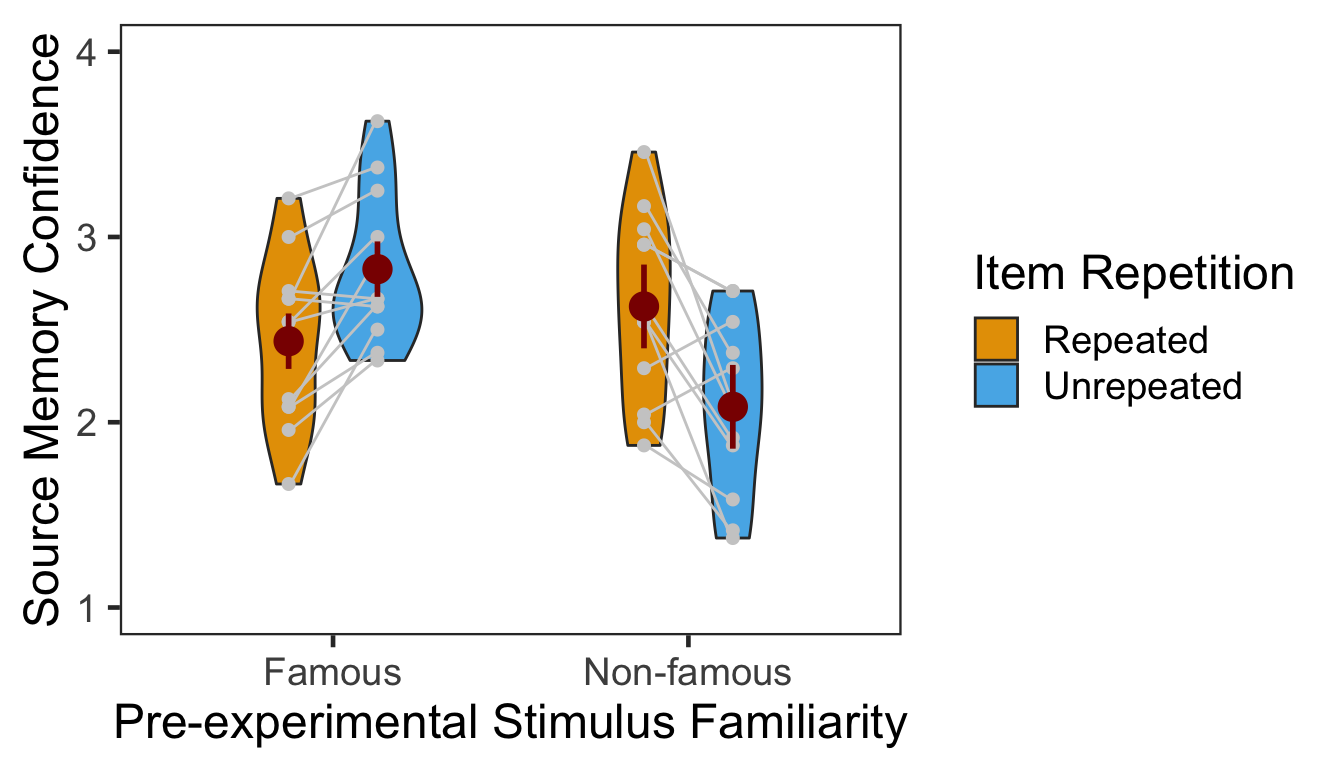### 3.2.1 ANOVA

Individuals’ mean confidence ratings were submitted to a 2x2 mixed design ANOVA with pre-experimental stimulus familiarity as a between-participants factor and item repetition as a within-participants factor.

p3.conf.aov <- aov_ez(id = "SID", dv = "Confidence", data = P3CFslong,
between = "Familiarity", within = "Repetition")
anova(p3.conf.aov, es = "pes") %>% kable(digits = 4)
num Df den Df MSE F pes Pr(>F)
Familiarity 1 22 0.3322 2.7875 0.1125 0.1092
Repetition 1 22 0.0910 0.7694 0.0338 0.3899
Familiarity:Repetition 1 22 0.0910 28.5428 0.5647 0.0000

The two-way interaction was significant while neither main effects reached significance. Next we performed two additional one-way repeated-measures ANOVAs as post-hoc analyses. The first table below presents the effect of item repetition for famous faces, and the second presents the same effect for non-famous faces.

ci95 <- P3CFswide %>% filter(Familiarity=="Famous") %>%
mutate(Diff = Unrepeated - Repeated) %>%
summarise(lower = mean(Diff) - qt(0.975,df=n()-1)*sd(Diff)/sqrt(n()),
upper = mean(Diff) + qt(0.975,df=n()-1)*sd(Diff)/sqrt(n()))

p3.conf.aov.r1 <- aov_ez(id = "SID", dv = "Confidence", within = "Repetition",
data = filter(P3CFslong, Familiarity == "Famous"))
anova(p3.conf.aov.r1, es = "pes") %>% kable(digits = 4)
num Df den Df MSE F pes Pr(>F)
Repetition 1 11 0.0555 16.3643 0.598 0.0019

In the famous face group, confidence ratings were higher for unrepeated than repeated faces. The 95% CI of difference between the means was [0.18, 0.6].

ci95 <- P3CFswide %>% filter(Familiarity=="Non-famous") %>%
mutate(Diff = Repeated - Unrepeated) %>%
summarise(lower = mean(Diff) - qt(0.975,df=n()-1)*sd(Diff)/sqrt(n()),
upper = mean(Diff) + qt(0.975,df=n()-1)*sd(Diff)/sqrt(n()))

p3.conf.aov.r2 <- aov_ez(id = "SID", dv = "Confidence", within = "Repetition",
data = filter(P3CFslong, Familiarity == "Non-famous"))
anova(p3.conf.aov.r2, es = "pes") %>% kable(digits = 4)
num Df den Df MSE F pes Pr(>F)
Repetition 1 11 0.1266 13.9077 0.5584 0.0033

In the non-famous face group, confidence ratings were higher for repeated than unrepeated faces. The 95% CI of difference between the means was [0.22, 0.86].

### 3.2.2 CLMM

The responses from a Likert-type scale are ordinal. Especially for the rating items with numerical response formats containing four or fewer categories, it is recommended to use categorical data analysis approaches, rather than treating the responses as continuous data (Harpe, 2015).

Here we employed the cumulative link mixed modeling (CLMM) using the clmm() of the package ordinal (Christensen, submitted). The specification of the full model was the same as the mixed() above. To determine the statistical significance, the likelihood ratio tests (LRT) compared models with or without the fixed effect of interest.

P3R <- P3
P3R$Confident = factor(P3R$Confident, ordered = TRUE)
P3R$SID = factor(P3R$SID)

cm.full <- clmm(Confident ~ Familiarity * Repetition + (Repetition|SID) + (1|ImgName), data=P3R)
cm.red1 <- clmm(Confident ~ Familiarity + Repetition + (Repetition|SID) + (1|ImgName), data=P3R)
cm.red2 <- clmm(Confident ~ Repetition + (Repetition|SID) + (1|ImgName), data=P3R)
cm.red3 <- clmm(Confident ~ 1 + (Repetition|SID) + (1|ImgName), data=P3R) 
cm.comp <- anova(cm.full, cm.red1, cm.red2, cm.red3)

data.frame(Effect = c("Familiarity", "Repetition", "Familiarity:Repetition"),
df = 1, Chisq = cm.comp$LR.stat[2:4], p = cm.comp$Pr(>Chisq)[2:4]) %>% kable()
Effect df Chisq p
Familiarity 1 0.4489511 0.5028335
Repetition 1 0.8270370 0.3631307
Familiarity:Repetition 1 19.3723293 0.0000108

The LRT revealed a significant two-way interaction. Neither main effects were significant. Next we performed pairwise comparisons as post-hoc analyses. As shown in the following table, the results of the pairwise comparisons were consistent with those from the ANOVA approach.

emmeans(cm.full, pairwise ~ Repetition | Familiarity)$contrasts %>% kable() contrast Familiarity estimate SE df z.ratio p.value Repeated - Unrepeated Famous -0.7610445 0.2402659 Inf -3.16751 0.0015375 Repeated - Unrepeated Non-famous 1.0914876 0.2384801 Inf 4.57685 0.0000047 Below is the plot of estimated marginal means, which were extracted from the fitted CLMM. The estimated distribution of confidence ratings shows the interaction between item repetition and pre-experimental stimulus familiarity. temp <- emmeans(cm.full,~Familiarity:Repetition|cut, mode="linear.predictor") temp <- rating.emmeans(temp) temp <- temp %>% unite(Condition, c("Familiarity", "Repetition")) ggplot(data = temp, aes(x = Rating, y = Prob, group = Condition)) + geom_line(aes(color = Condition), size = 1.2) + geom_point(aes(shape = Condition, color = Condition), size = 4, fill = "white", stroke = 1.2) + scale_color_manual(values=c("#E69F00", "#E69F00", "#56B4E9", "#56B4E9")) + scale_shape_manual(name="Condition", values=c(21,24,21,24)) + labs(y = "Response Probability", x = "Rating") + expand_limits(y=0) + scale_y_continuous(limits = c(0, 0.4)) + scale_x_discrete(labels = c("1" = "1(Guessed)","4"="4(Sure)")) + theme_minimal() + theme(text = element_text(size=18))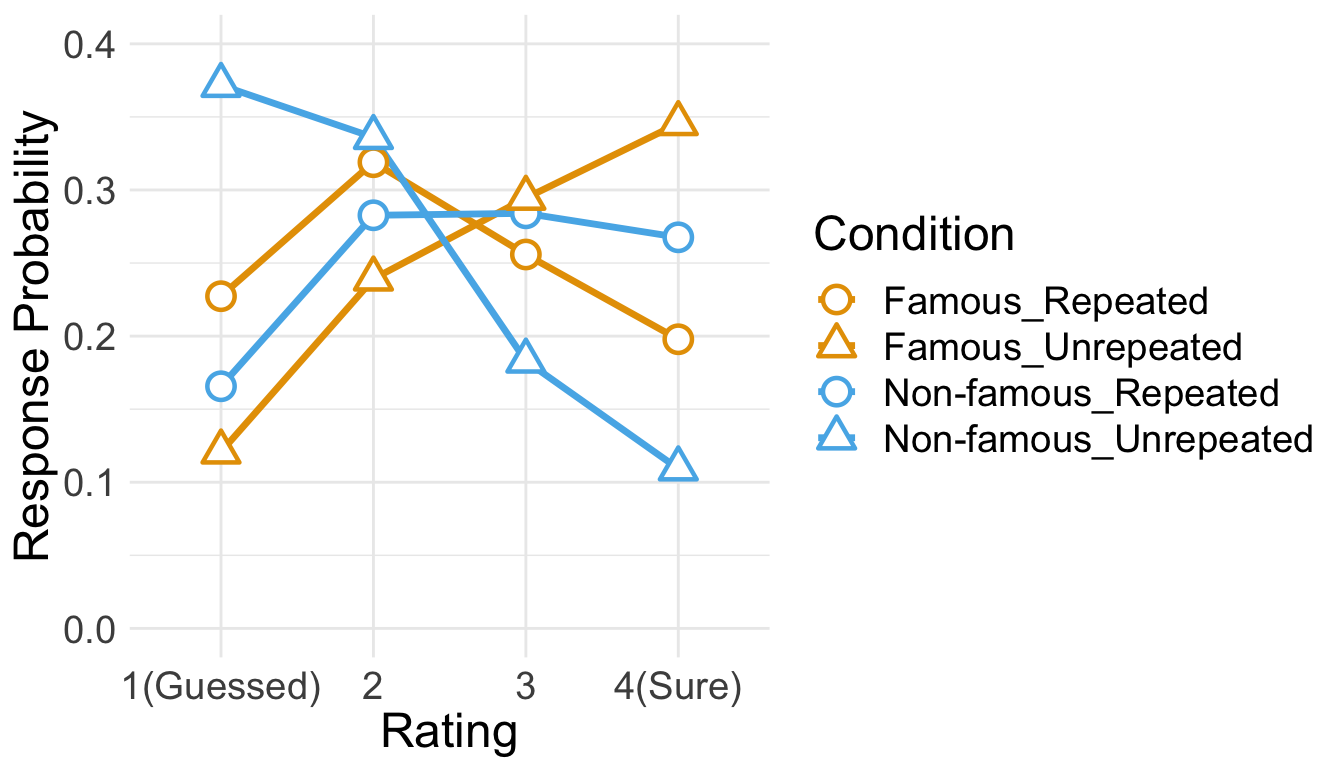ww.conf.t.r1 <- t.test(Confidence ~ Repetition, filter(P3CFslong, Familiarity == "Famous"), paired = TRUE) sort(abs(ww.conf.t.r1$conf.int))[1:2] # 95% CI of difference between means
##  0.1772996 0.6004781

ww.conf.t.r2 <- t.test(Confidence ~ Repetition, filter(P3CFslong, Familiarity == "Non-famous"), paired = TRUE)
sort(abs(ww.conf.t.r2$conf.int))[1:2] # 95% CI of difference between means ##  0.2219825 0.8613508 ## 3.3 RT Only RTs in correct trials were analyzed. Before analysis, we first removed RTs either shorter than 200ms or longer than 10s. Then, from the RT distribution of each condition, RTs beyond 3 s.d. from the mean were additionally removed. cP3 <- P3 %>% filter(Corr==1) sP3 <- cP3 %>% filter(RT > 200 & RT < 10000) %>% group_by(SID) %>% nest() %>% mutate(lbound = map(data, ~mean(.$RT)-3*sd(.$RT)), ubound = map(data, ~mean(.$RT)+3*sd(.$RT))) %>% unnest(lbound, ubound) %>% unnest(data) %>% ungroup() %>% mutate(Outlier = (RT < lbound)|(RT > ubound)) %>% filter(Outlier == FALSE) %>% select(SID, Familiarity, Repetition, RT, ImgName) 100 - 100*nrow(sP3)/nrow(cP3) ##  2.882883 This trimming procedure removed 2.88% of correct RTs. Since the overall source memory accuracy was not high, only small numbers of correct trials were available after trimming. The following table summarizes the numbers of trials submitted to subsequent analyses. No participant had more than 25 trials per condition. In the non-famous group, half of the participants had less than 10 valid trials per condition. sP3 %>% group_by(SID, Familiarity, Repetition) %>% summarise(NumTrial = length(RT)) %>% ungroup %>% group_by(Familiarity, Repetition) %>% summarise(Avg = mean(NumTrial), Med = median(NumTrial), Min = min(NumTrial), Max = max(NumTrial)) %>% ungroup %>% kable() Familiarity Repetition Avg Med Min Max Famous Repeated 11.583333 12.0 4 19 Famous Unrepeated 15.083333 14.5 11 21 Non-famous Repeated 10.333333 9.5 6 15 Non-famous Unrepeated 7.916667 7.5 3 15 den1 <- ggplot(cP3, aes(x=RT)) + geom_density() + theme_bw(base_size = 18) + theme(panel.grid.major = element_blank(), panel.grid.minor = element_blank(), axis.text.y = element_blank(), axis.ticks.y = element_blank()) den2 <- ggplot(sP3, aes(x=RT)) + geom_density() + theme_bw(base_size = 18) + labs(x = "Trimmed RT") + theme(panel.grid.major = element_blank(), panel.grid.minor = element_blank(), axis.text.y = element_blank(), axis.ticks.y = element_blank()) den1 + den2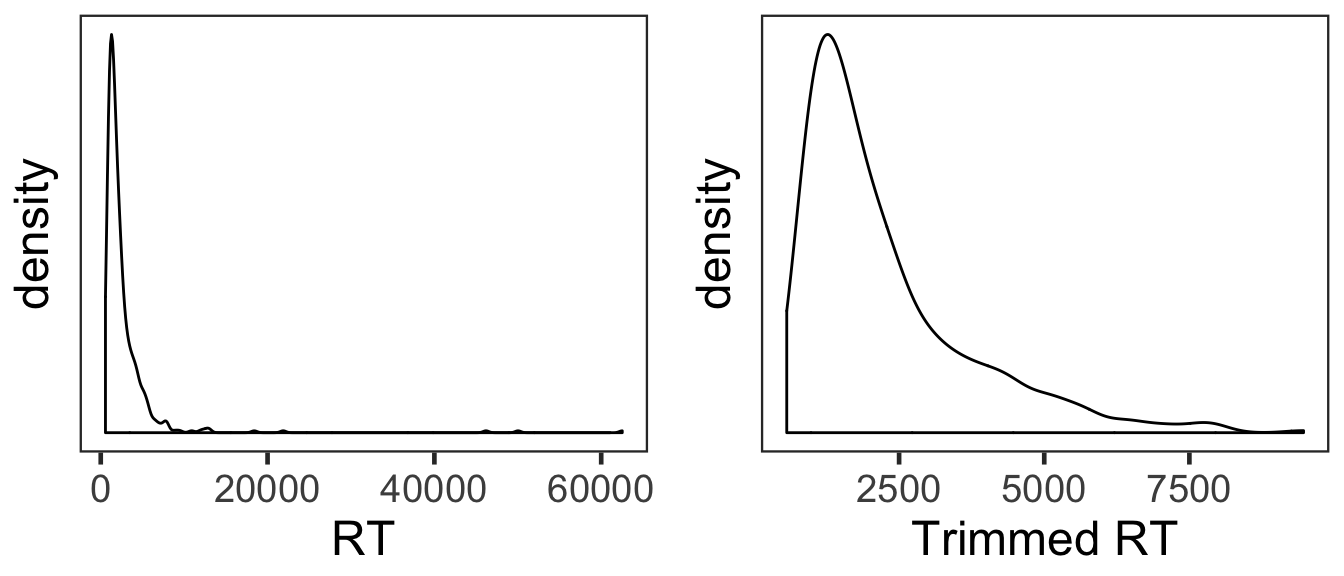The overall RT distribution was highly skewed even after trimming. Given limited numbers of RTs and its skewed distribution, any results from the current RT analyses should be interpreted with caution and preferably corroborated with other measures. We calculated the mean and s.d. of individual participants’ mean RTs. The overall pattern of RTs across the conditions was consistent with that of source memory accuracy and confidence ratings. In the following plot, red points and error bars represent the means and 95% within-participants CIs. P3RTslong <- sP3 %>% group_by(SID, Familiarity, Repetition) %>% summarise(RT = mean(RT)) %>% ungroup() P3RTg <- P3RTslong %>% group_by(Familiarity, Repetition) %>% summarise(M = mean(RT), SD = sd(RT)) %>% ungroup() P3RTg %>% kable() Familiarity Repetition M SD Famous Repeated 2355.159 1102.2211 Famous Unrepeated 2051.237 710.7829 Non-famous Repeated 2434.308 996.1972 Non-famous Unrepeated 2580.130 1117.5506 # wide format, needed for geom_segments. P3RTswide <- P3RTslong %>% spread(key = Repetition, value = RT) # group level, needed for printing & geom_pointrange # Rmisc must be called indirectly due to incompatibility between plyr and dplyr. P3RTg$ci <- Rmisc::summarySEwithin(data = P3RTslong, measurevar = "RT", idvar = "SID",
withinvars = "Repetition", betweenvars = "Familiarity")$ci P3RTg$RT <- P3RTg$M ggplot(data=P3RTslong, aes(x=Familiarity, y=RT, fill=Repetition)) + geom_violin(width = 0.5, trim=TRUE) + geom_point(position=position_dodge(0.5), color="gray80", size=1.8, show.legend = FALSE) + geom_segment(data=filter(P3RTswide, Familiarity=="Famous"), inherit.aes = FALSE, aes(x=1-.12, y=filter(P3RTswide, Familiarity=="Famous")$Repeated,
xend=1+.12, yend=filter(P3RTswide, Familiarity=="Famous")$Unrepeated), color="gray80") + geom_segment(data=filter(P3RTswide, Familiarity=="Non-famous"), inherit.aes = FALSE, aes(x=2-.12, y=filter(P3RTswide, Familiarity=="Non-famous")$Repeated,
xend=2+.12, yend=filter(P3RTswide, Familiarity=="Non-famous")\$Unrepeated),
color="gray80") +
geom_pointrange(data=P3RTg,
aes(x = Familiarity, ymin = RT-ci, ymax = RT+ci, group = Repetition),
position = position_dodge(0.5), color = "darkred", size = 1, show.legend = FALSE) +
scale_fill_manual(values=c("#E69F00", "#56B4E9"),
labels=c("Repeated", "Unrepeated")) +
labs(x = "Pre-experimental Stimulus Familiarity",
y = "Response Times (ms)",
fill='Item Repetition') +
theme_bw(base_size = 18) +
theme(panel.grid.major = element_blank(),
panel.grid.minor = element_blank())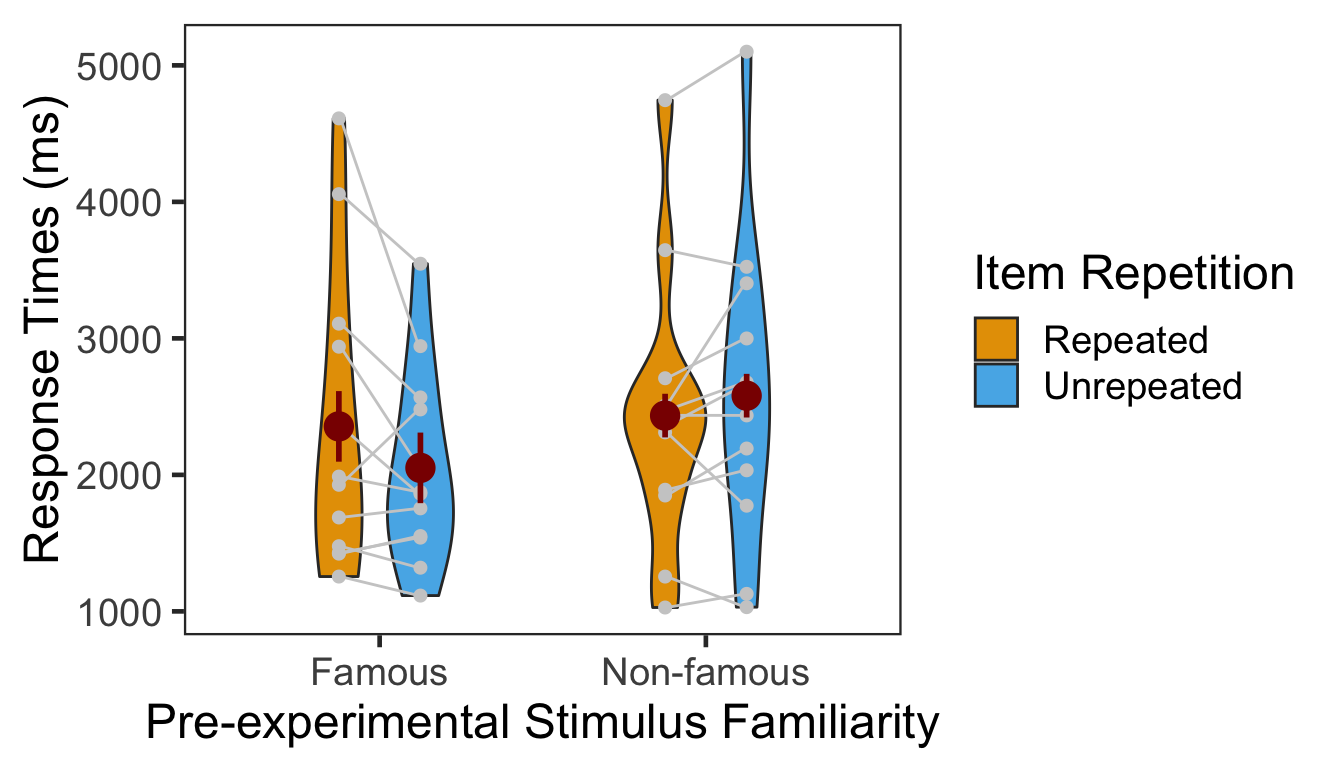### 3.3.1 ANOVA

Individuals’ mean RTs were submitted to a 2x2 mixed design ANOVA with pre-experimental stimulus familiarity as a between-participants factor and item repetition as a within-participant factor.2

p3.rt.aov <- aov_ez(id = "SID", dv = "RT", data = sP3,
between = "Familiarity", within = "Repetition")
anova(p3.rt.aov, es = "pes") %>% kable(digits = 4)
num Df den Df MSE F pes Pr(>F)
Familiarity 1 22 1866257.4 0.5943 0.0263 0.4490
Repetition 1 22 114458.6 0.6551 0.0289 0.4269
Familiarity:Repetition 1 22 114458.6 5.3016 0.1942 0.0311

The two-way interaction was significant. We then performed two one-way repeated-measures ANOVAs as post-hoc analyses. The first table below presents the effect of item repetition for famous faces, and the second table presents the same effect for non-famous faces.

p3.rt.aov.r1 <- aov_ez(id = "SID", dv = "RT", within = "Repetition",
data = filter(sP3, Familiarity == "Famous"))
anova(p3.rt.aov.r1, es = "pes") %>% kable(digits = 4)
num Df den Df MSE F pes Pr(>F)
Repetition 1 11 165604.1 3.3466 0.2333 0.0946

The effect of item repetition was not significant in the famous condition.

p3.rt.aov.r2 <- aov_ez(id = "SID", dv = "RT", within = "Repetition",
data = filter(sP3, Familiarity == "Non-famous"))
anova(p3.rt.aov.r2, es = "pes") %>% kable(digits = 4)
num Df den Df MSE F pes Pr(>F)
Repetition 1 11 63313.16 2.0151 0.1548 0.1835

The effect of item repetition was not significant in the non-famous condition, either.

# 4 Session Info

sessionInfo()
## R version 3.5.2 (2018-12-20)
## Platform: x86_64-apple-darwin15.6.0 (64-bit)
## Running under: macOS High Sierra 10.13.6
##
## Matrix products: default
## BLAS: /Library/Frameworks/R.framework/Versions/3.5/Resources/lib/libRblas.0.dylib
## LAPACK: /Library/Frameworks/R.framework/Versions/3.5/Resources/lib/libRlapack.dylib
##
## locale:
##  en_US.UTF-8/en_US.UTF-8/en_US.UTF-8/C/en_US.UTF-8/en_US.UTF-8
##
## attached base packages:
##  parallel  stats     graphics  grDevices utils     datasets  methods
##  base
##
## other attached packages:
##   klippy_0.0.0.9500    patchwork_0.0.1      RVAideMemoire_0.9-73
##   ggbeeswarm_0.6.0     ordinal_2019.3-9     emmeans_1.3.3
##   afex_0.23-0          lme4_1.1-21          Matrix_1.2-17
##  car_3.0-2            carData_3.0-2        knitr_1.22
##  forcats_0.4.0        stringr_1.4.0        dplyr_0.8.0.1
##  tibble_2.1.1         ggplot2_3.1.0        tidyverse_1.2.1
##  Rmisc_1.5            plyr_1.8.4           lattice_0.20-38
##  pacman_0.5.1
##
## loaded via a namespace (and not attached):
##   nlme_3.1-137      lubridate_1.7.4   httr_1.4.0
##   numDeriv_2016.8-1 tools_3.5.2       backports_1.1.3
##   utf8_1.1.4        R6_2.4.0          vipor_0.4.5
##  lazyeval_0.2.2    colorspace_1.4-1  withr_2.1.2
##  tidyselect_0.2.5  curl_3.3          compiler_3.5.2
##  cli_1.1.0         rvest_0.3.2       xml2_1.2.0
##  sandwich_2.5-0    labeling_0.3      scales_1.0.0
##  mvtnorm_1.0-10    digest_0.6.18     foreign_0.8-71
##  minqa_1.2.4       rmarkdown_1.12    rio_0.5.16
##  pkgconfig_2.0.2   htmltools_0.3.6   highr_0.8
##  generics_0.0.2    zoo_1.8-5         jsonlite_1.6
##  zip_2.0.1         magrittr_1.5      fansi_0.4.0
##  Rcpp_1.0.1        munsell_0.5.0     abind_1.4-5
##  ucminf_1.1-4      stringi_1.4.3     multcomp_1.4-10
##  yaml_2.2.0        MASS_7.3-51.3     grid_3.5.2
##  crayon_1.3.4      haven_2.1.0       splines_3.5.2
##  hms_0.4.2         pillar_1.3.1      boot_1.3-20
##  estimability_1.3  reshape2_1.4.3    codetools_0.2-16
##  glue_1.3.1        evaluate_0.13     data.table_1.12.0
##  modelr_0.1.4      nloptr_1.2.1      cellranger_1.1.0
##  gtable_0.3.0      assertthat_0.2.1  xfun_0.6
##  openxlsx_4.1.0    xtable_1.8-3      broom_0.5.1
##  coda_0.19-2       survival_2.44-1.1 lmerTest_3.1-0
##  beeswarm_0.2.3    TH.data_1.0-10

1. One additional participant in the famous face group was removed from analysis for not responding in 87.5% of trials in the item-source association phase.

2. We additionally tested several GLMMs on source memory RTs since GLMMs with relevant link functions were supposed to be better than the conventional ANOVA in dealing with such limited, unbalanced and skewed data as the current RTs (Lo & Andrews, 2015). Some models adopted non-linear transformations of RTs (such as -1000/RT or log(RT)) and others assumed an inverse Gaussian or Gamma distribution and a linear relationship (identity link function) between the predictors and RTs. None of the models, however, converged onto a stable solution.